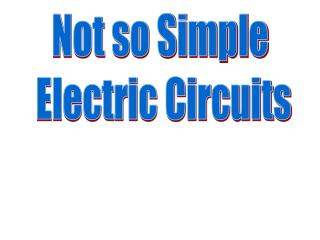Download PresentationNot so Simple Electric Circuits

# Not so Simple Electric Circuits - PowerPoint PPT PresentationDownload Presentation## Not so Simple Electric Circuits

- - - - - - - - - - - - - - - - - - - - - - - - - - - E N D - - - - - - - - - - - - - - - - - - - - - - - - - - -
##### Presentation Transcript

1. Not so Simple Electric Circuits

2. Why can a bird stand on a high voltage wire and not get shocked? Because there is no Potential difference between his feet. Will either bird get shocked now? Yes, the one over the light bulb, because a potential difference exists between his feet.

3. A word about Electrical Safety… Ground plugs. One end of the ground plug is connected to the appliance cover. The wall jack which receives the ground plug is connected to the ground, so any charge leaking onto the appliance will drain to ground not you. If not… You are the path of least resistance..

4. One milliampere: tingling sensationTen milliamperes: nerves and muscles overloaded200 milliamperes: potentially fatal; heart fibrillation500 -1000 milliamperes: not necessarily fatal; heart will restartOne ampere or more: burn alive

5. As more and more appliances are added to a circuit in parallel the resistance decreases and the current increases. Too much current can result in an overload and a possible fire. Fuses and Circuit breakers are used to prevent overload, by limiting the amount of current that can flow through a circuit. A fuse uses a thin metal wire or ribbon that melts when current through it is too high. These must be replaced.

6. Houses today use CIRCUIT BREAKERS. These do not need to be replaced and use a bimetallic strip that trips a switch. Brass expands more than steel As temperature increases, the bimetallic strip arcs to the left,settles into groove, and the spring pulls the metal bar down breaking the circuit.

7. How can we solve complex circuits? That is circuits that have both parallel and series components? We will use Equivalent Resistance …

8. Equivalent Resistance simplifies a circuit by replacing resistor sets with a single EQUAL resistor… For example: The 8Ω and 4Ω resistors are in series so could be replaced with a 12Ω resistor The 6Ω and 3Ω resistors are in parallel. They can be replaced with a single 2 Ω resistor. The 12 Ω and 2 Ω resistors are in series and could be replaced with a single 14 Ω resistor.

9. Example: The total applied voltage to the circuit in the figure is 12 V and the resistances R1, R2 and R3are 4, 3 and 6 Ω respectively. a. Determine the equivalent resistance of the circuit. R2 and R3 are in parallel (RP) Rp= 2 Ω RP and R1 are in series Req= 4 + 2 = 6 Ω

10. The total applied voltage to the circuit in the figure is 12 V and the resistances R1, R2 and R3 are 4, 3 and 6 Ω respectively. (b) What is the total current? I = V/R 12V/6Ω = 2 A (c) Find the current through and the voltage across each resistor. R1: I = 2 A (series) so V=IR= (2 A)(4 Ω)= 8V The remaining voltage in the circuit is 12 V – 8 V = 4 V which means that V2 and V3 are 4 V since they are in parallel. I = V/R I2 = 4V / 3Ω = 1.33 A I3 = 4V / 6Ω = 0.67 A